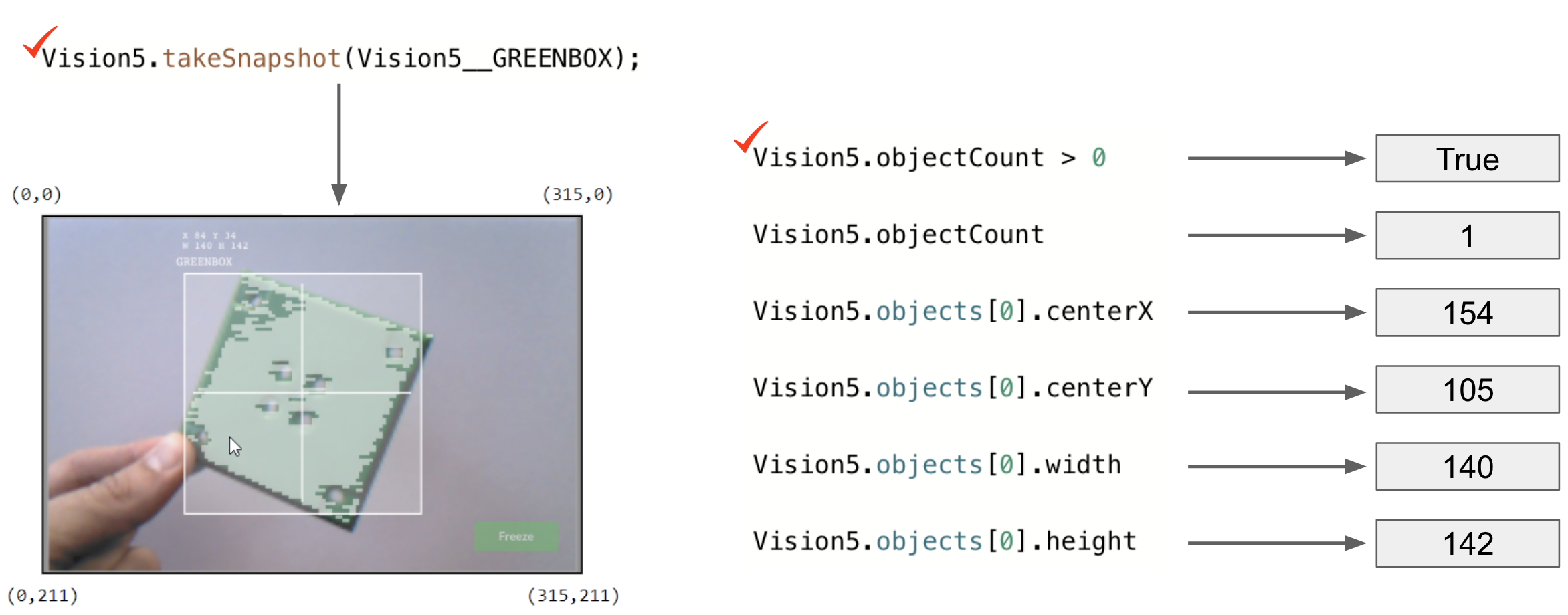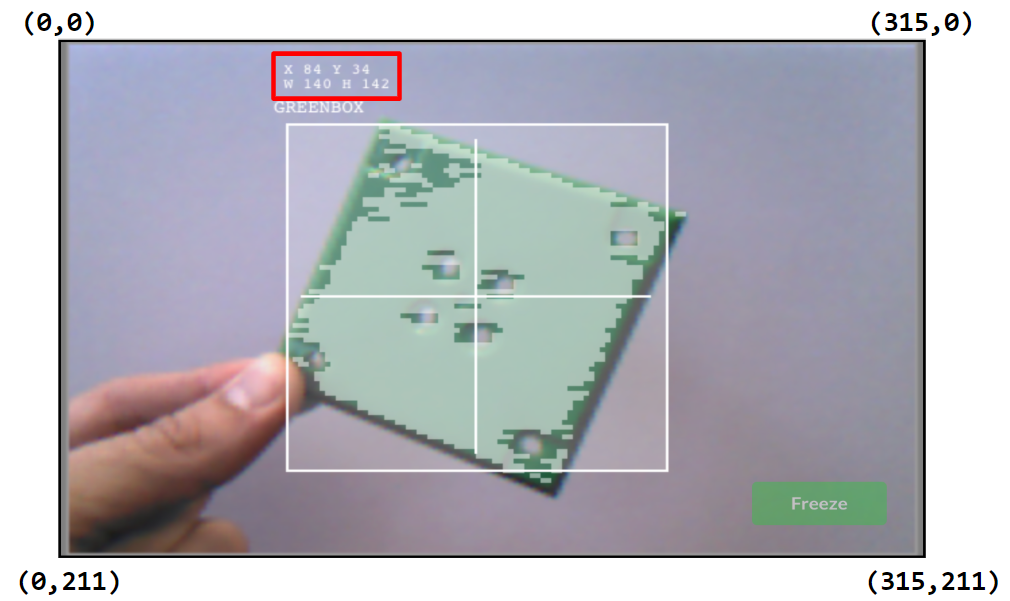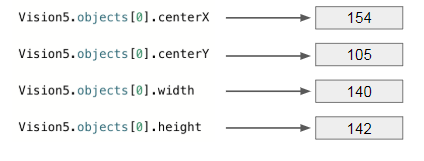STEMLabs V5
Vision Sensor Teacher

Teacher Portal

# Prepare for the Vision Data Challenge - Text-basedTeacher Toolbox - The Purpose of this Activity

The Vision Sensor provides a variety of data that can then be used in projects. The Sensing instruction allows the user to have the project take snapshots, decide if the object exists, decide how many exist, determine the object's center X and Y coordinates within the Vision Sensor's snapshot, and determine the object's width and height in pixels within the snapshot. This activity will introduce all of the related instruction necessary for collecting that information in preparation for the Vision Data Challenge.
The following is an outline of Rethink's Vision Data Challenge:

• Review a complete data set of information collected from the Vision Sensor's Sensing instruction.

• Complete a partial data set of information collected from the Vision Sensor about a different snapshot.

• Create a data set based on a snapshot and the Vision Sensor's Sensing instructions.

### The Vision Sensor's Sensing Instructions

VEXcode V5 has Sensing instruction for the Vision Sensor. The first two you already used in the Play section to take a snapshot and to check if the object exists.

In the figure below, you see that the snapshot captured the GREENBOX snapshot. The object, GREENBOX, was identified in the snapshot and so the answer of whether it exists is TRUE.

Let's look at these other Sensing instructions and what their values tell us.• The object count instruction tells us how many GREENBOX objects are in the snapshot. Here, there is only 1 detected.

• The center X value tells us whether the GREENBOX object is to the left or right of the robot's center point. Remember, the Vision Sensor should be mounted in the middle of the robot facing forward and so the snapshot's view is the robot's view.

• If center X is greater than 157.5, the object is to the right of the robot's center point.

• If center X is less than 157.5, the object is to the left of the robot's center point.

• The center Y value tells us whether the GREENBOX is higher or lower than the robot's center point.

• If center Y is greater than 105.5, the object is lower than the robot's center point.

• If center Y is less than 105.5, the object is higher than the robot's center point.

• The width and height values tell us how close the GREENBOX is to the robot.

• The same-sized object will be larger in width and height as it gets closer to the robot.Teacher Toolbox - Why this reading?

The Help information within VEXcode V5 also provides information about the instructions but here, the data being collected are contextualized as to what they specifically tell the user about the object in the snapshot.

Notes:

• The centerX and centerY values of the entire snapshot are used for determining whether the object is to the left/right or above/below the robot's center point. They are calculated by dividing the total number of pixels on that axis by two (e.g., centerX of snapshot = 315 / 2 = 157.5).

We can assume the center point of the robot is the same as the center point of the Vision Sensor's snapshot because the Vision Sensor should be mounted in the center of the robot and facing forward. The position of the Vision Sensor on the robot's build and the degree to which the Vision Sensor might be angled downward need to be taken into account when judging the position of the object relative to the robot's (or Vision Sensor's) center point.

• The Y values increase downward within the snapshot. Make sure students recognize that before moving on to the next part.

### How are the center X and center Y values calculated?

The values are calculated based on the coordinates within the snapshot. The width and height of the object are already calculated.

The Vision Sensor tracks the X and Y values of the upper left corner of the object. Below, those coordinates are (84, 34).The center X and center Y values can be calculated based off of the coordinates of the upper left corner (84, 34), and the width (W 140) and height (H 142) values provided.• centerX = 140/2 + 84 = 154

• centerX = half the width of the object added to its leftmost X coordinate

• centerY = 142/2 + 34 = 105

• centerY = half the height of the object added to its topmost Y coordinateTeacher Toolbox - Concluding this page

Make sure that students understand the math involved in finding the center X and center Y values. They will need it for the activity on the next page.

Ask how the (84, 34) and (W 140, H 142) values relate to the coordinates provided in the corners of the snapshot. Students should recognize that the entire snapshot is mapped onto a coordinate plane based on the number of pixels. The X values range from 0 to 315 (316 pixels wide) and the Y values range from 0 to 211 (212 pixels tall). The object's coordinates and size are based on how many pixels the object takes up along those axes.